# Mathematics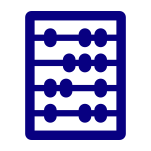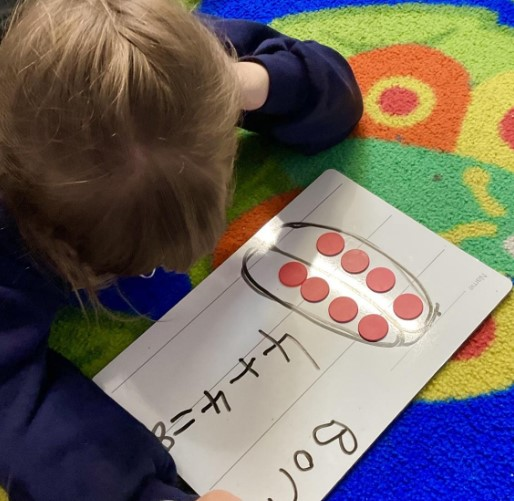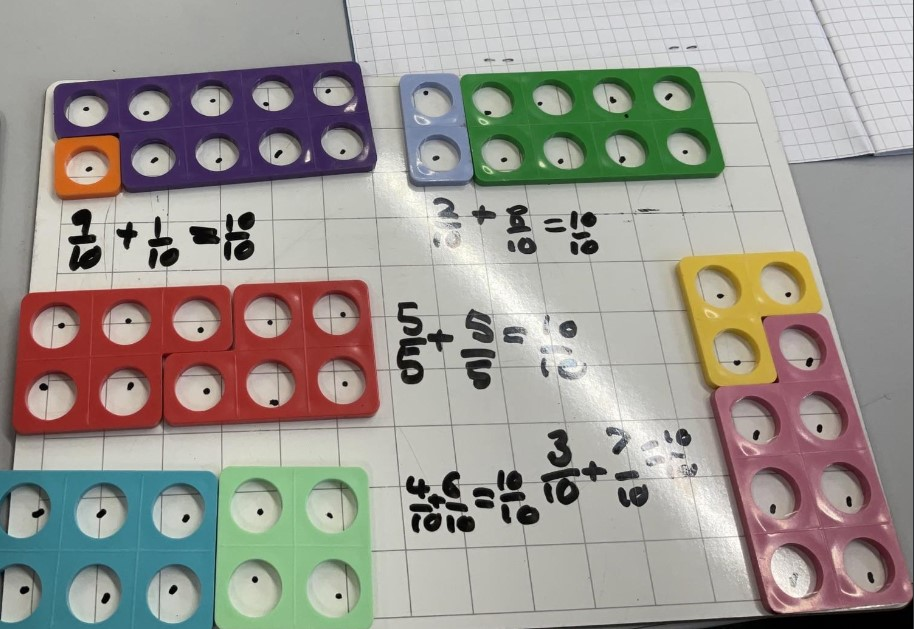## AIMS

The national curriculum for mathematics aims to ensure that all pupils:

•  become fluent in the fundamentals of mathematics, including through varied and frequent practice with increasingly complex problems over time, so that pupils develop conceptual understanding and the ability to recall and apply knowledge rapidly and accurately.
• reason mathematically by following a line of enquiry, conjecturing relationships and generalisations, and developing an argument, justification or proof using mathematical language
• can solve problems by applying their mathematics to a variety of routine and nonroutine problems with increasing sophistication, including breaking down problems into a series of simpler steps and persevering in seeking solutions

## TEACHING METHODS

##### Concrete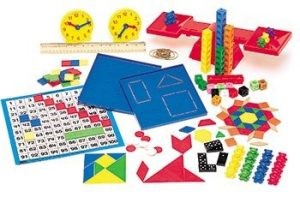##### Pictorial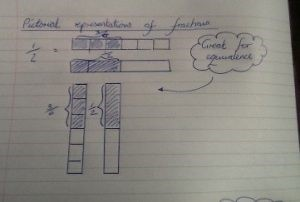##### Abstract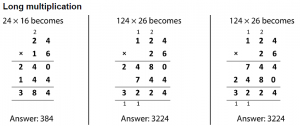At Wateringbury, we follow the prin​ciples of maths mastery using the ‘White Rose’ resources. The White Rose overviews have number at their heart. A large proportion of time is spent reinforcing number to build competency.  They also provide plenty of opportunities to build reasoning and problems elements into the curriculum.

We use concrete, pictorial and abstract approaches to achieving mathematical understanding. This approach is a highly effective way of teaching that develops a deep and sustainable understanding of maths. With the ambitious expectations of the National Curriculum, we aim to ensure that our children understand every mathematical topic, are able to explain their methods and can make connections between concepts.  Mastery of maths gives children the ability to reason and talk in depth about their work. All year groups follow this format with reasoning and problem-solving at the heart.

In each of the strands, pupils are taught to work mentally, to develop paper and pencil skills, and to understand how to apply what they know to real-life situations.

#### Times Tables and Artihmetic Fluency

We have developed a consistent approach to the teaching of calculations for each group to enable each child to become fluent in the fundamentals of maths through varied and frequent practice so that pupils develop the ability to recall and apply knowledge rapidly and accurately.

From June 2022 Year 4 children will participate in statutory online multiplication tables checks.

## WHY DO WE TEACH THIS WAY?

• To promote a confident, positive attitude towards the learning and use of mathematics, making it an enjoyable experience;
• To promote confidence and competence with numbers and the number system;
• To promote the ability to solve problems through connecting ideas, making decisions and applying mathematical skills in a range of contexts, including other subjects such as science;
• To promote mathematical reasoning by following a line of enquiry, developing an argument and making justifications using mathematical language;
• To promote a practical understanding of the ways in which information is gathered, presented and used;
• To promote the exploration of features of shape and space and develop measuring skills in a range of contexts;
• To understand the importance of mathematics in everyday use, especially in relation to essential life skills, such as telling the time and understanding money;
• To foster positive attitudes towards mathematics by developing pupil’s confidence, independence, persistence and co-operations skills.

Mathematics threshold concepts:

• Know and use numbers

This concept involves understanding the number system and how they are used in a wide variety of mathematical ways.

This concept involves understanding both the concepts and processes of addition and subtraction.

• Multiply and divide

This concept involves understanding both the concepts and processes of multiplication and division.

• Use fractions

This concept involves understanding the concept of part and whole and ways of calculating using it.

• Understand the properties of shapes

This concept involves recognising the names and properties of geometric shapes and angles.

• Describe position, direction and movement

This concept involves recognising various types of mathematical movements.

• Use measures

This concept involves becoming familiar with a range of measures, devices used for measuring and calculations.

• Use statistics

This concept involves interpreting, manipulating and presenting data in various ways.

• Use algebra

This concept involves recognising mathematical properties and relationships using symbolic representations.

Essential characteristics of Wateringbury mathematicians:

• An understanding of the important concepts and an ability to make connections within mathematics.
• A broad range of skills in using and applying mathematics.
• Fluent knowledge and recall of number facts and the number system.
• The ability to show initiative in solving problems in a wide range of contexts, including the new or unusual.
• The ability to think independently and to persevere when faced with challenges, showing a confidence of success.
• The ability to embrace the value of learning from mistakes and false starts.
• The ability to reason, generalise and make sense of solutions.
• Fluency in performing written and mental calculations and mathematical techniques.
• A wide range of mathematical vocabulary.
• A commitment to and passion for the subject.